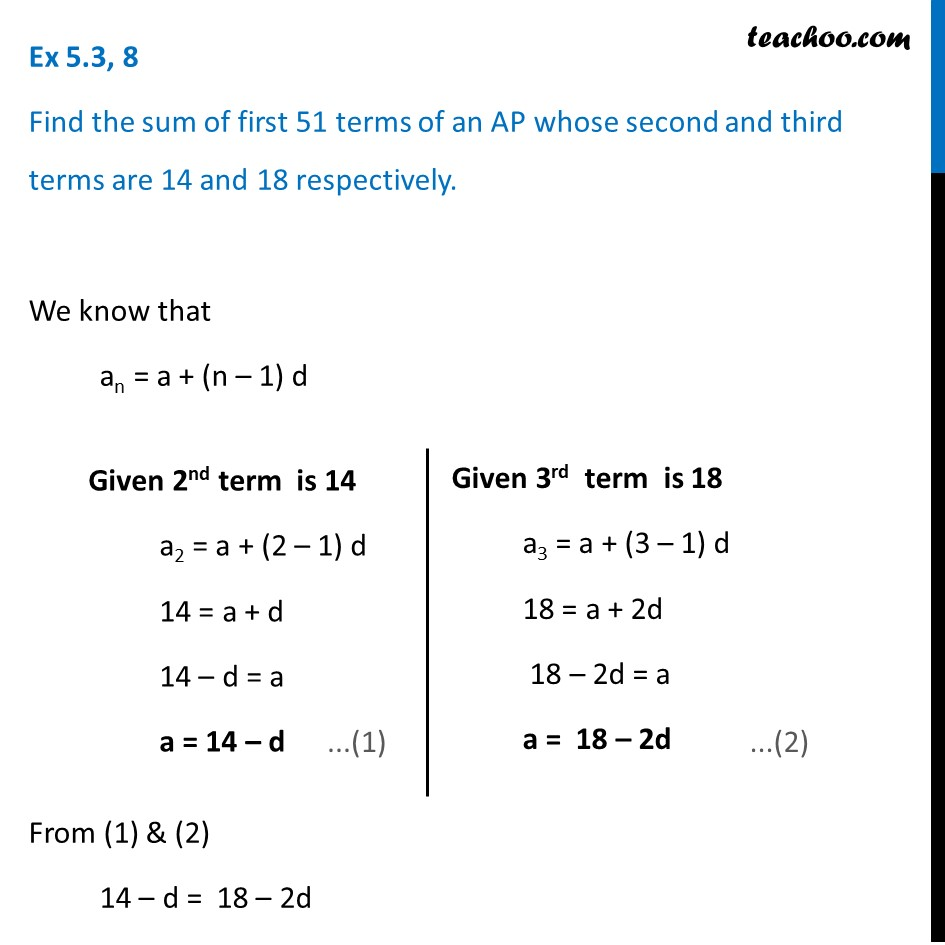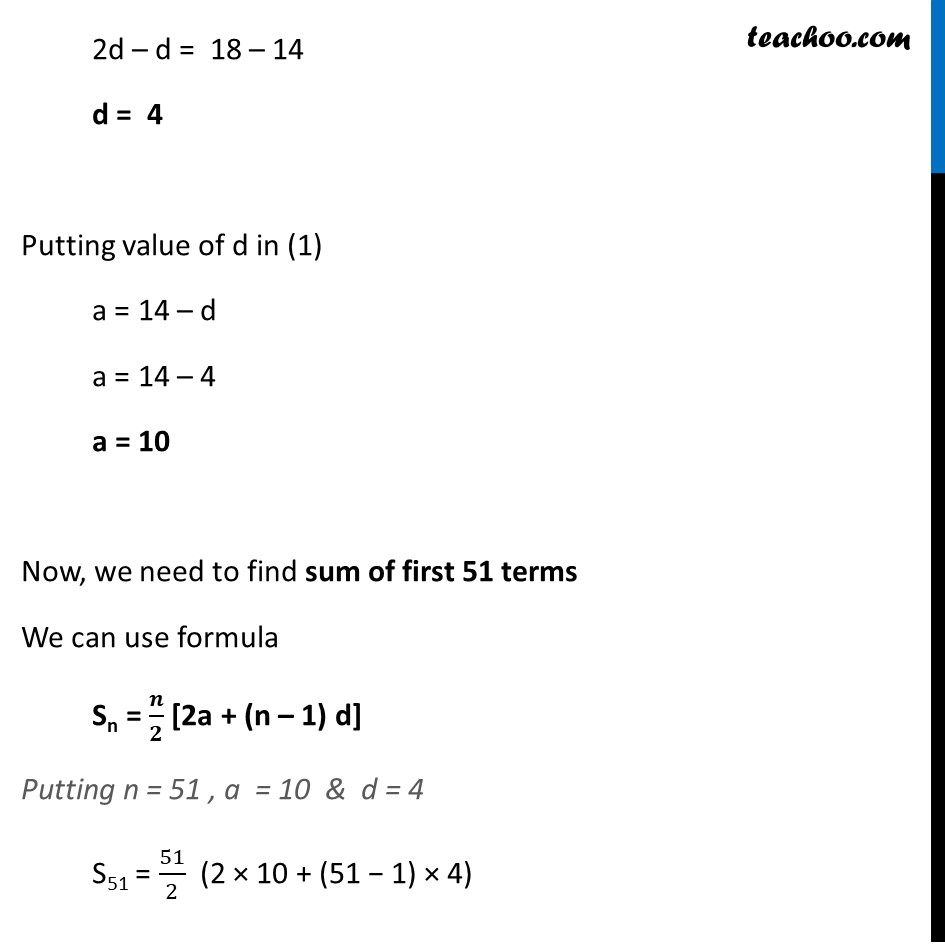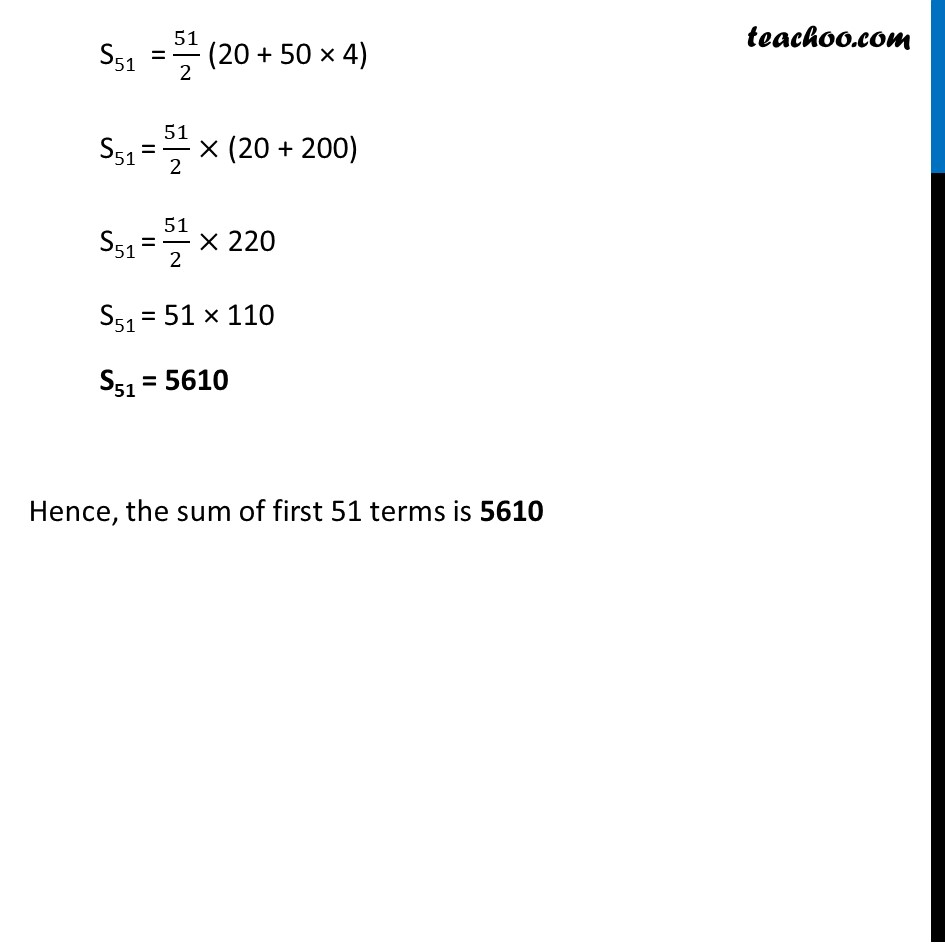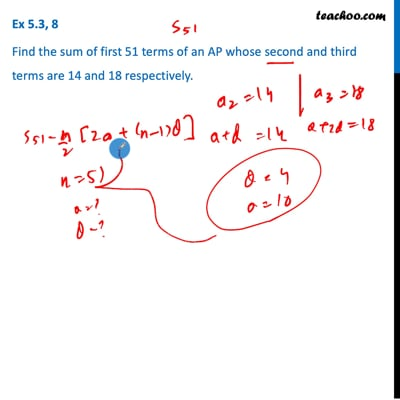Ex 5.3

Chapter 5 Class 10 Arithmetic Progressions
Serial order wiseThis video is only available for Teachoo black users

Learn in your speed, with individual attention - Teachoo Maths 1-on-1 Class

### Transcript

Ex 5.3, 8 Find the sum of first 51 terms of an AP whose second and third terms are 14 and 18 respectively. We know that an = a + (n – 1) d From (1) & (2) 14 – d = 18 – 2d Given 2nd term is 14 a2 = a + (2 – 1) d 14 = a + d 14 – d = a a = 14 – d Given 3rd term is 18 a3 = a + (3 – 1) d 18 = a + 2d 18 – 2d = a a = 18 – 2d 2d – d = 18 – 14 d = 4 Putting value of d in (1) a = 14 – d a = 14 – 4 a = 10 Now, we need to find sum of first 51 terms We can use formula Sn = 𝒏/𝟐 [2a + (n – 1) d] Putting n = 51 , a = 10 & d = 4 S51 = 51/2 (2 × 10 + (51 − 1) × 4) S51 = 51/2 (20 + 50 × 4) S51 = 51/2 × (20 + 200) S51 = 51/2 × 220 S51 = 51 × 110 S51 = 5610 Hence, the sum of first 51 terms is 5610